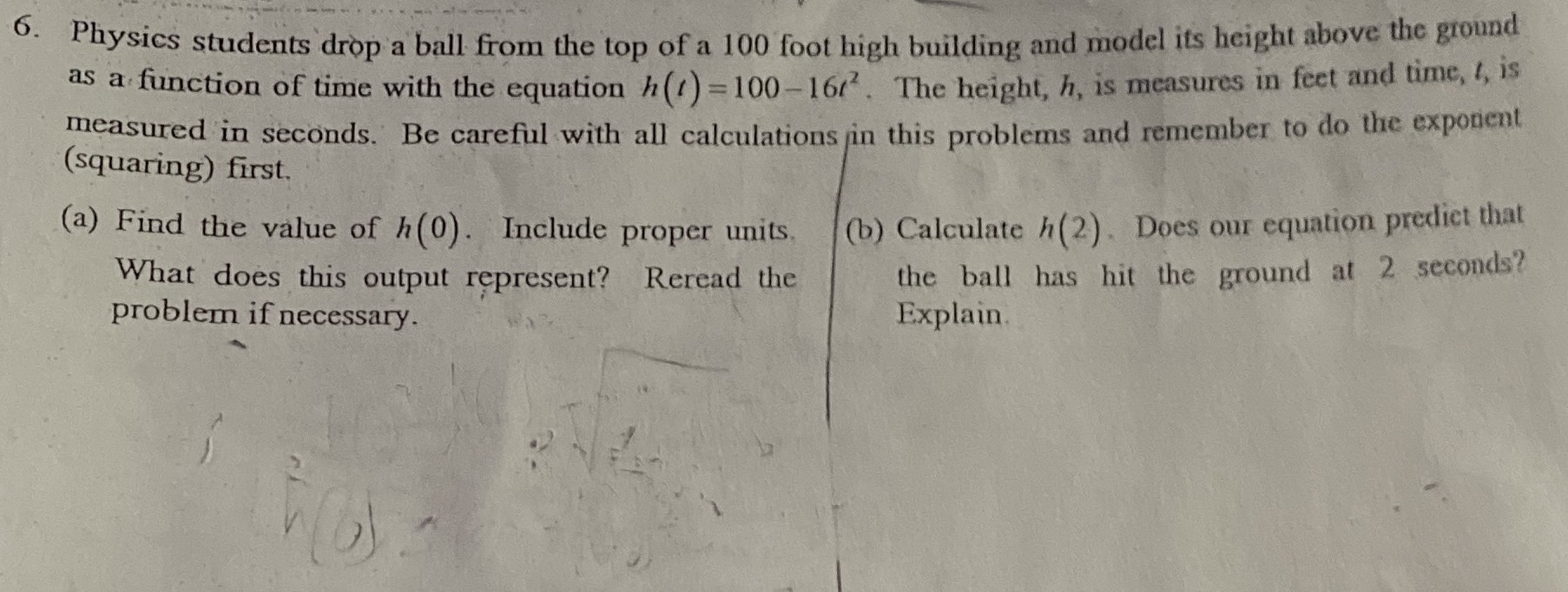### Still have math questions?

Algebra
Question6. Physics students drop a ball from the top of a $$100$$ foot high building and model its height above the ground as a function of time with the equation $$h ( t ) = 100 - 16 t ^ { 2 }$$ . The height, $$h$$ , is measures in feet and time, $$t$$ , is measured in seconds. Be careful with all calculations in this problems and remember to do the exponent (squaring) first.

(a) Find the value of $$h ( 0 )$$ . Include proper units. What does this output represent? Reread the problem if necessary.

(b) Calculate $$h ( 2 )$$ . Does our equation predict that  the ball has hit the ground at $$2$$ seconds? Explain.